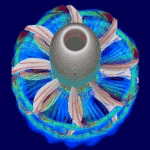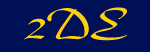Go to.... Home About Me -Resume -Diploma Thesis Aerospace -Turbofan Directory -Turbofan Analysis -CFD -NozzleFloQ1D -2DE Travels & Gallery Music Links[Home] [About Me] [Aerospace] [Travels & Gallery] [Music] [Links]Presented here is an example of a complete solution of a subsonic internal channel flow over a 10% bump.
This test case, a commonly used reference case, is run in 2DE by specifying an inflow total pressure of 1e5 N/m^2, an inflow total Temperature of 300K, an outflow static pressure of 8e4 N/m^2 and a pressure ratio across the channel of 1. Using a single block, structured euler grid of 96x32 cells this case serves to demonstrate the convergence improvements to be achieved with the use of multigrid. Initially a single grid run is performed, which exhibits an extremely slow rate of convergence. This problem is solved though the use of multigrid, as the results for a run using various 2-, 3- and 4-grid cycles demonstrate. For all three computations the solver run is done using local time-stepping, a CFL number of 7.5, implicit residual smoothing with variable coefficients scaled with an epsilon of 0.8, and 2nd and 4th order artificial viscosity terms scaled with factors of 0.5 and 1/64 respectively. For the multigird cases the coarse grid corrections are smoothed using a constant coefficient of 0.01 on all grid levels.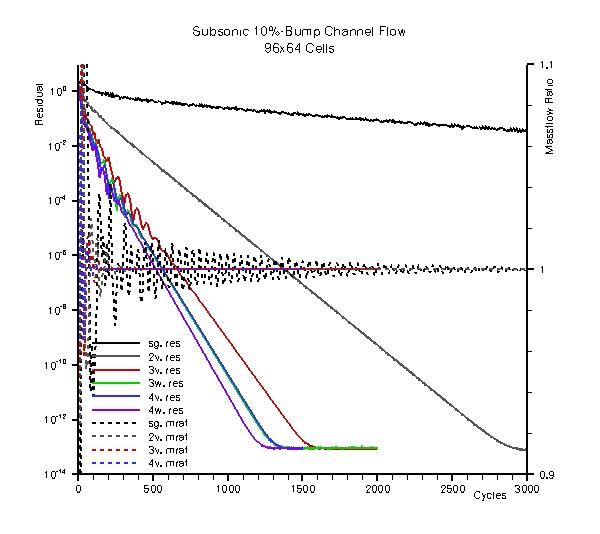Comparative plot of the density residuals and the mass flow ratio across the channel for the various solver runs. The single-grid computations show a very poor rate of convergence in terms of the density residual and still exhibits fluctuations in the mass flow ratio after 3000 cycles. A significant improvement can already be achieved by using a 2v-multigrid cycle, which leads to a bottoming out of the density residual after 3000 cycles. Using a 3v-multigrid cycle results in a fully converged solution after 1600 cycles, while machine accuracy is achieved after 1400 cycles for both a 3w- and a 4v-cycle. For the 4w-cycle a fully converged solution can be reached after 1300 cycles, which is the lowest number of iterations of all of the multigrid cycles tested.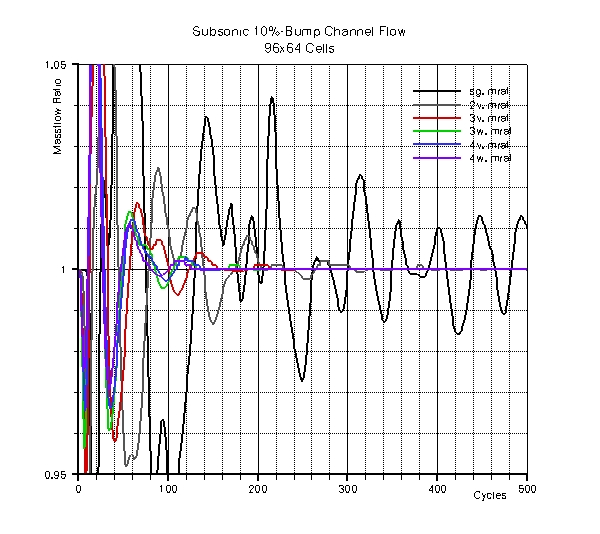The benefit of running this case with multigrid also becomes evident when looking at the evolution of the mass flow-ratio across the channel during the first 500 cycles of the various computations. The single grid run shows strong oscillations which can be damped out much earlier using multigrid acceleration. Much like the situation for the overall rate of convergence described above, using more grid levels as well as using a w- instead of a v-cycle tends to speed up the damping out of oscillations in the mass flow-ratio, with the 4w-multigrid cycle leading to a constant value after only about 150 cycles.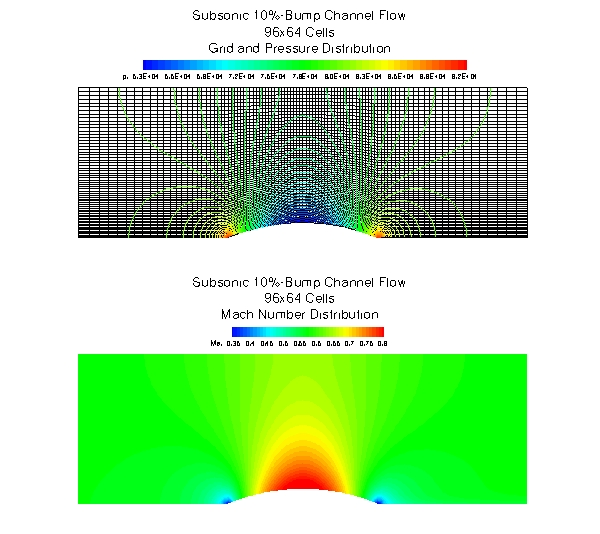The 2D-contour plot of the mach number distribution shows completely subsonic flow through the channel, with only a slight acceleration on the top of the bump. The top image shows iso-lines of pressure superimposed on the grid of the finest level used in this study.
 Case Cycles (to 1e-13) Mass flow [kg/s] Mass flow Ratio Runtime [s] Ave. Time per Cycle [s] Time to Convergence [s] SG 3000 (-) 190.8 1 449 0.149667 - 2V 3000 (2896) 190.9 1 630 0.21 608.16 3V 2000 (1578) 190.9 1 461 0.2305 363.729 3W 2000 (1362) 190.9 1 566 0.283 385.446 4V 1500 (1352) 190.9 1 346 0.230667 311.861784 4W 1500 (1253) 190.9 1 364 0.242667 304.061751

This table lists details pertaining to the convergence and run times for the various cases. The value in parentheses in the cycle column is the iteration at which the density residual drops below a value of 1e-13 for the first time. This convergence criteria is somewhat arbitrary but does allow for a fairly accurate comparison of the time-to-convergence as the final density residual fluctuates around a value of 9e-14 for all cases. For all cases the physically relevant properties mass flow and mass flow ratio have reached identical values at convergence. Of interest is the time to convergence, which is a product of the average runtime per cycle and the iteration number at which the density residual reaches 1e-13. It shows - with one notable exception - that the improved convergence rate of w-cycles over v-cycles and of increasing the number of multigrid levels also translates to an overall increase in efficiency, i.e. runtime to convergence. However the 4w-cycle does seem to be close to the optimum in terms of efficiency, as the improvement over the 4v-cycle is relatively small. The notable exception mentioned is the 3w-cycle, which is slightly more expensive than the 3v-cycle in terms of compute time. A possible explanation for this is that here three rather expensive Runge-Kutta cycles on the 2nd finest grid level need to be performed per cycle, while only two are required for the 3v-cycle. The extra RK-cycle on the 3rd finest grid level for the 4w-cycle versus the 4v-cycle on the other hand seems to be less costly and more effective in improving convergence, making the time to convergence for the 4w-cycle the quickest of the cases tested here.